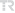## Investigation of the Behaviour of Volterra Integral Equations with Random Effects

Gümüşhane Üniversitesi Fen Bilimleri Dergisi, vol.10, no.1, pp.205-216, 2020 (Peer-Reviewed Journal)• Publication Type: Article / Article
• Volume: 10 Issue: 1
• Publication Date: 2020
• Doi Number: 10.17714/gumusfenbil.586796
• Journal Name:
• Journal Indexes: TR DİZİN (ULAKBİM)
• Page Numbers: pp.205-216
• Recep Tayyip Erdoğan University Affiliated: Yes

#### Abstract

In this study, random Volterra integral equations obtained by transforming components of deterministic Volterraintegral equations to random variables are analysed. Beta, Normal (Gaussian), Gamma, Geometric and Uniformdistributions are used to investigate the random behaviour of the solutions for Volterra integral equations under randomeffects. The random version of Differential Transformation Method (RDTM) is used to obtain an approximation to thesolution of the random Volterra integral equation. Using the approximate solutions, approximate expected values andapproximate variances are calculated. Some integro-differential equations, obtained by using random components withthe above mentioned distributions, are solved as numerical examples. Results are obtained in MAPLE and shown ingraphs. It is seen that random Differential Transformation Method is effective for the examination of random Volterraintegral equations. Comparison of the solutions is given to underline the accuracy of the method.